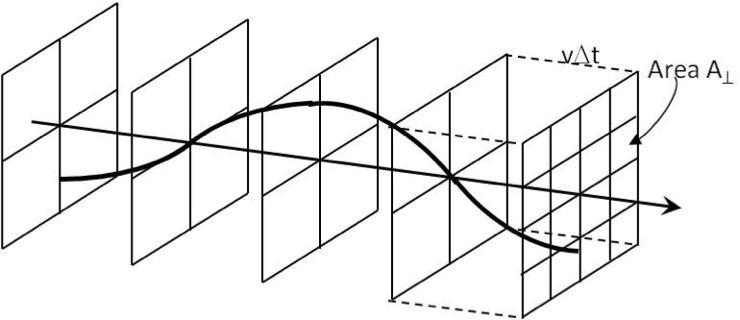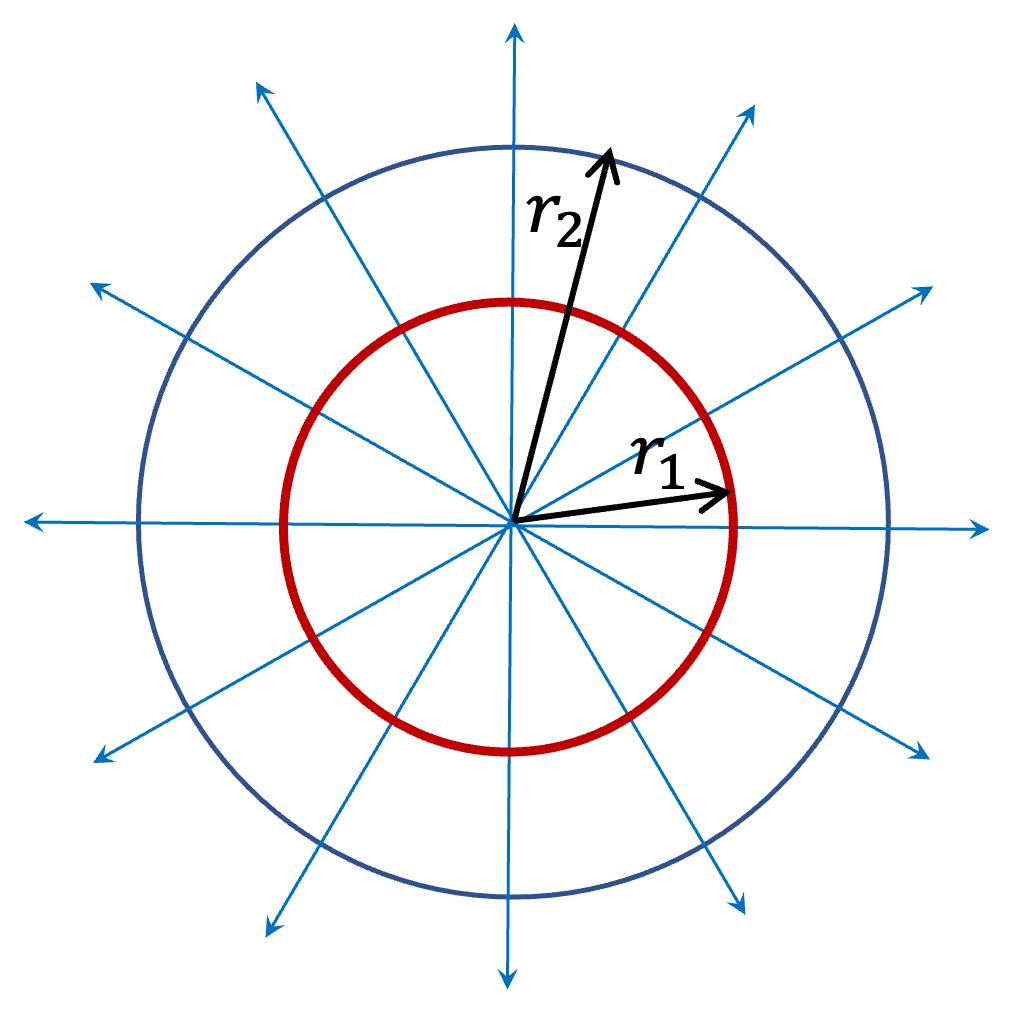## Section14.7Energy, Power, and Intensity

### Subsection14.7.1Energy Transported by Plane Wave

All waves transport energy without an actual transport of material. For concreteness, as shown in Figure 14.7.1 consider a wave of frequency $f\text{,}$ wavelength $\lambda\text{,}$ and amplitude $D$ on a taut string with tension $T$ and mass per unit length $\mu\text{.}$ We denote the amplitude of the displacement of string particles by letter $D$ rather than letter $A$ since the later will be used for area below. Supposing the wave is moving in the positive $x$ axis direction wave will have the following expression.

\begin{equation*} \psi = D \cos\left( \frac{2\pi}{\lambda} x - 2\pi f t\right). \end{equation*}Figure 14.7.1. A wave passing through a cross-sectional area; intensity of the wave is defined as average power passing per unit are perpendicular to the wave direction. The wave shown here has speed $v\text{.}$ The energy contained in the box of area $A_{\perp}$ and height $v\Delta t$ pass through $A_{\perp}$ in time $\Delta t\text{.}$

In Figure 14.7.1, the energy of the wave will be contained in the motion (kinetic energy) and the deformation (potential energy). Let us figure out the energy contained within a distance $v\Delta t$ of the plane labeled $A_\perp$ in the figure. Since each part of the string executes simple harmonic motion at frequency $f$ of the wave, we can use formulas for energy derived in the simple harmonic motion to get some ideas about energy involved here. Let $K$ stand for effective spring constant (we use capital $K$ to distinguish it from wavenumber $k$) and $M$ the mass of string in length $v\Delta t\text{,}$ where $v$ is the wave speed. Let $V$ denote maximum speed of motion of particles of string. Thus, average energy within a length $v\Delta t$ of the string can be estimated to be

\begin{equation} E_\text{av} = \frac{1}{2} K D^2 = \frac{1}{2} M V^2\tag{14.7.1} \end{equation}

Additionally, by analogy with simple harmonic oscillator, we can replace $V$ by

\begin{equation} V = \omega D= 2\pi f D.\tag{14.7.2} \end{equation}

Using this we find

\begin{equation} K = M \omega^2 = 4\pi^2 M f^2.\tag{14.7.3} \end{equation}

Therefore,

\begin{equation} E_\text{av} = \frac{1}{2} K D^2 = 2 \pi^2 M f^2 D^2.\tag{14.7.4} \end{equation}

This energy will pass any point of string in time $\Delta t\text{.}$ Therefore, the average rate of flow of energy, i.e., average power $P\text{,}$ in the string will be

\begin{align} P_\text{av} \amp = \frac{E_\text{av}}{\Delta t} = \frac{2 \pi^2 M f^2 D^2}{\Delta t}, \notag\\ \amp = \frac{2 \pi^2 M v f^2 D^2}{v\Delta t} = 2 \pi^2 \mu v f^2D^2, \notag\\ \amp = 2 \pi^2 \mu \lambda f^3 D^2, \label{eq-power-in-wave-on-a-string}\tag{14.7.5} \end{align}

This says that the rate at which energy is transported by a wave is proportional to the square of the amplitude of the wave.

\begin{equation} P_\text{av} \propto D^2.\tag{14.7.6} \end{equation}

It is of interest to know how “concentrated” the power is in any region. Therefore, we define a property called intensity of the wave by dividing power by the area of the surface the wave is flowing through, here it will be $A_\perp\text{.}$

\begin{equation} I = \frac{P_\text{av}}{A_\perp} = \frac{2 \pi^2 \mu \lambda f^3 D^2}{A_\perp}.\label{eq-definition-of-intensity}\tag{14.7.7} \end{equation}

Imporant observation here again is that intensity of a wave is proportional to the square of the amplitude.

\begin{equation} I \propto D^2.\tag{14.7.8} \end{equation}

For a plane wave intensity does not change from one location to another or with time. This is not the case for spherical wave as we will see below.

Note that $D$ in Eq. (14.7.7) has the units of length since it corresponds to displacement in its derivation. The unit of intensssity will be

\begin{equation*} \text{unit of } I = \frac{\text{kg}}{\text{m}} \times \frac{1}{\text{s}^3} \frac{\text{m}^2}{\text{m}^2} = \frac{\text{W}}{\text{m}^2}, \end{equation*}

where $\text{W}$ is Watts or $\text{J/s}\text{.}$

### Subsection14.7.2Intensity of a Spherical Wave

Imagine a point source that emits a wave isotropically in all directions. Sun is a good example of this type of a source. Since the wave spreads out in a three-dimensional space isotropically, i.e., without a preferred direction, the wave will be a spherical wave.

Let $P_0$ be the rate at which energy is input in the wave at the center. Then, in a duration $\Delta t\text{,}$ an energy equal to $\Delta E = P_0\Delta t$ will pass through both the surfaces at $r=r_1$ and $r=r_2\text{.}$ Dividing this energy by time $\Delta t$ and the areas of corresponding surfaces we get the following for the intensities at the two surfaces.\begin{align} \amp I_1 = \dfrac{P_0 \Delta t }{\Delta t \times 4\pi r_1^2},\label{eq-intensity-1-full}\tag{14.7.9}\\ \amp I_1 = \dfrac{P_0 \Delta t }{\Delta t \times 4\pi r_1^2}.\label{eq-intensity-2-full}\tag{14.7.10} \end{align}

Dividing these equations, we get

\begin{equation} \frac{I_1}{I_2} = \frac{r_2^2}{r_1^2}. \tag{14.7.11} \end{equation}

This suggests a simple rule to remember in the case of spherically spreading wave.

\begin{equation} I \propto \frac{1}{r^2}.\tag{14.7.12} \end{equation}

A plane wave in the air of density $1.1\text{ kg/m}^3$ is given by the following function.

\begin{equation*} \psi(x,y,z,t) = 4\text{ cm } \cos\left(10\, z - 200\, t + \frac{\pi}{2} \right), \end{equation*}

where $z$ is in $\text{m}\text{,}$ $t$ in $\text{sec}\text{.}$

(a) What are the intensities of the wave at the following points (i) $(0,0,0)$ and (ii) $(1,1,1)\text{?}$

(b) How much energies will pass through an area $3\text{ cm}^2$ in $2\text{ sec}$ if the area is in the (i) $xy$-plane, (ii) $yz$-plane and (iii) $xz$-plane?

Hint

(a) Read off $D,\ k, \omega$ from wave function and use $\mu=\rho A_\perp\text{.}$ (b) Only area perpendicular to the wave direction will have energy flow.

(a) (i) $500\text{ W/m}^2\text{,}$ (ii) Same as (i). (b) (i) $2.1\text{ J}\text{,}$ (ii) $0\text{,}$ (iii) $0\text{.}$

Solution 1 (a)

(a) First we note that intensity of a plane wave is independent of position. Therefore, we will get the same anser for the two cases (i) and (ii).

From the wave function we note the following quantities we need for the intensity formula.

\begin{align*} \amp D = 4\text{ cm} = 0.04\text{ m},\\ \amp k = 10\text{ m}^{-1} \longrightarrow \lambda = 0.2\ \pi \text{ m},\\ \amp \omega = 200\text{ s}^{-1} \longrightarrow f = \frac{100}{\pi} \text{ Hz}, \end{align*}

The linear density $\mu=\rho A_\perp$ as you can show by equating mass in a volume in two different ways, one using linear density $\mu$ and another using regular density $\rho\text{,}$ i.e., $\mu L = \rho A_\perp L\text{.}$ Using Eq. (14.7.7), we get

\begin{align*} I \amp = \frac{2 \pi^2 \mu \lambda f^3 D^2}{A_\perp} = 2 \pi^2 \rho\lambda f^3 D^2\\ \amp = 2 \pi^2 \times 1.1 \times 0.2\,\pi \times (100/\pi)^3\times 0.04^2 = 3500\text{ W/m}^2. \end{align*}
Solution 2 (b)

(b) From the argument of cosine in the wave function, the wave is moving towards positive $z$ axis. Therefore, no power will flow through any area through which wave does not pass. This makes $E=0$ for (ii) and (iii). In case of (i), energy through area $A_\perp$ in time $\Delta t$ will be

\begin{equation*} E = I A_\perp \Delta t. \end{equation*}

Therefore, we will get

\begin{equation*} E = 3500\frac{\text{J}}{\text{s.m}^2} \times 3\times 10^{-4}\text{ m}^2\times 2\text{ s} = 2.1\text{ J}. \end{equation*}

A spherical wave is given by the following function.

\begin{equation*} \psi(x,y,z,t) = \frac{A}{r} \cos\left(10\, r - 200\, t \right), \end{equation*}

where $r$ is in $\text{m}\text{,}$ $t$ in $\text{sec}$ and $\psi$ in $\text{m}\text{.}$ Here $r$ is spherical radial coordinate from the source at the origin. The intensity of the wave at $r=0.25\text{ m}$ from the origin is $10\text{ W/m}^2\text{.}$

(a) What are the intensities of the wave at the following points (i) $(0,0,1)\text{,}$ (ii) $(0,0,2)\text{,}$ and $(1,1,1)\text{?}$

(b) How much energies will pass through an area $3\text{ cm}^2$ in $2\text{ sec}$ if the area is part of spherical shells at radial distances (i) $2\text{ cm}\text{,}$ (ii) $4\text{ cm}\text{,}$ and (iii) $8\text{ cm}$ from the origin.

Hint

(a) Use $I\propto 1/r^2\text{.}$ (b) Use $E = I A t\text{.}$

(a) (i) $0.625\text{ W/m}^2\text{,}$ (ii) $0.156\text{ W/m}^2\text{,}$ (iii) $0.208\text{ W/m}^2\text{.}$ (b) (i) $0.94\text{ J}\text{,}$ (ii) $0.23\text{ J}\text{,}$ (iii) $0.06\text{ J}\text{.}$

Solution 1 (a)

(a) Intensity of a spherical wave drops off as inverse square of distance from

\begin{align*} \amp (i)\ \ \ I = \frac{10\text{ W/m}^2 \times (0.25\text{ m})^2}{ (1.0\text{ m})^2} = 0.625 \text{ W/m}^2.\\ \amp (ii)\ \ I = \frac{10\text{ W/m}^2 \times (0.25\text{ m})^2}{ (2.0\text{ m})^2} = 0.156 \text{ W/m}^2. \\ \amp (iii)\ I = \frac{10\text{ W/m}^2 \times (0.25\text{ m})^2}{ (\sqrt{3}\text{ m})^2} = 0.208 \text{ W/m}^2. \end{align*}
Solution 2 (b)

(b) The wave is moving ouward radially. Therefore, it will pierce each of the area perpendicularly. Hence, we can just umultiply the intensity at that radial distance with area given and the duration given. The intensities at these distances are

\begin{align*} \amp (i)\ \ \ I = \frac{10\text{ W/m}^2 \times (0.25\text{ m})^2}{ (0.02\text{ m})^2} = 1563 \text{ W/m}^2.\\ \amp (ii)\ \ I = \frac{10\text{ W/m}^2 \times (0.25\text{ m})^2}{ (0.04\text{ m})^2} = 391 \text{ W/m}^2. \\ \amp (iii)\ I = \frac{10\text{ W/m}^2 \times (0.25\text{ m})^2}{ (0.08\text{ m})^2} = 98 \text{ W/m}^2. \end{align*}

Therefore, we will get

\begin{align*} \amp (i)\ \ \ E = 1563 \times 0.0003\times 2 = 0.94\text{ J}.\\ \amp (ii)\ \ E = 0.23\text{ J}. \\ \amp (iii)\ E = 0.06\text{ J}. \end{align*}

A spherical mechanical wave traveling in salt water of density $1050\text{ kg/m}^3$ is given by the following function.

\begin{equation*} \psi(x,y,z,t) = \frac{A}{r} \cos\left(10\, r - 200\, t \right), \end{equation*}

where $r$ is in $\text{m}\text{,}$ $t$ in $\text{sec}$ and $\psi$ in $\text{m}\text{.}$ Here $r$ is spherical radial coordinate from the source at the origin. The intensity of the wave at $r=0.25\text{ m}$ from the origin is $120\text{ W/m}^2\text{.}$

Find the value of constant $A$ in the wave function.

Hint

Start with $I = \frac{2 \pi^2 \mu \lambda f^3 D^2}{A_\perp}$ and replace $\mu$ by $\rho 4\pi r^2$ and $D= A/r\text{.}$ Note the unit of $A$ will be $\text{m}^2\text{.}$

$7.54\times 10^{-5}\text{ m}^2\text{.}$

Solution

We will use $I = \frac{2 \pi^2 \mu \lambda f^3 D^2}{A_\perp}$ with $D=A/r$ to find the value of $A\text{.}$ We note that the wave will pass through a spherical surface of radius $r\text{.}$ Hence,

\begin{equation*} A_\perp = 4\pi r^2. \end{equation*}

Therefore, we have the following for intensity at $r\text{.}$

\begin{equation*} I = \frac{\pi \mu \lambda f^3 A^2}{2 r^4}. \end{equation*}

By equating mass in $r$ to $r+\Delta r$ in two ways, one using linear density $\mu\Delta r$ and another by regular density $\rho \times A\Delta r$ with $A=4\pi r^2\text{,}$ we get

\begin{equation*} \mu = 4\pi r^2 \rho. \end{equation*}

Therefore

\begin{equation*} I = \frac{2\pi^2 \rho \lambda f^3 A^2}{r^2}. \end{equation*}

Replace $\lambda$ by $2\pi/k$ and $f$ by $\omega/2\pi$ to get

\begin{equation*} I = \pi \rho \frac{\omega^3}{2k} \frac{A^2}{r^2}. \end{equation*}

Solving for $A$ we get

\begin{align*} A \amp = \sqrt{ \frac{2 k r^2 I}{\pi \rho\omega^3} } \\ \amp = \sqrt{ \frac{2\times 10 \times 0.25^2 \times 120}{\pi\times 1050\times 200^3} } = 7.54\times 10^{-5}\text{ m}^2. \end{align*}